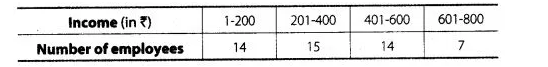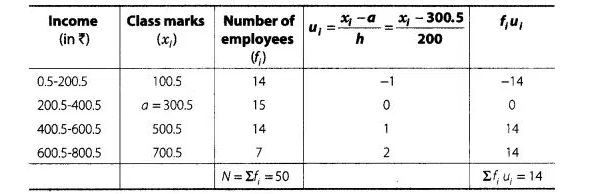# The daily income of a sample

Question:

The daily income of a sample of 50 employees are tabulated as follows.Find the mean daily income of employees.

Solution:

Since, given data is not continuous, so we subtract 0.5 from the lower limit and add 0.5 in the upper limit of each class.

Now we first, find the class mark xi, of each class and then proceed as follows$\therefore \quad$ Assumed mean, $a=300.5$

Class width, $h=200$

and total observations, $N=50$

By step deviation method,

Mean $=a+h \times \frac{1}{N} \times \sum_{i=1}^{5} f_{i} u_{i}$

$=300.5+200 \times \frac{1}{50} \times 14$

$=300.5+56=356.5$